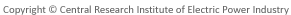#※ PDFのファイルサイズが大きい場合には、ダウンロードに時間がかかる場合がございます。　ダウンロードは1回のクリックで開始しますので、ダウンロードが完了するまで、複数回のクリックはなさらないようご注意願います。

## 電力中央研究所　報告書（電力中央研究所報告）

### 報告書データベース　詳細情報

Y03020

#### タイトル（英文）

The Impacts of Decreasing CO2 Emission by Changing Tax System in Japan

#### 概要 （英文）

This study quantitatively analyzes the economic impacts of imposing environment tax on Japanese economy and energy demand by using the long-term economic forecasting system of CRIEPI. Four cases are set according to the differences in imposing method of environment tax and returning method of tax revenue, and the impacts of environment tax in each case are analyzed. The results of the analyses are as follows: (1) In the Basic Carbon Tax Case where the reduction of income tax is assumed, the tax rates of 8,400 yen per t-C and 42,000 yen per t-C become necessary in 2010 and 2025, respectively. These are quite smaller than those of previous study (CRIEPI Report Y01007, in 2001), because the CO2 emission in the Reference Case where the carbon tax is not imposed has decreased greatly on account of low economic growth and the effects of various measures for CO2 emission mitigation taken recently.(2) Necessary carbon tax levels become higher if the rates of existing energy taxes are exempted or abolished. On the other hand, the total revenue of energy taxes and a carbon tax is smaller than that of the Basic Carbon Tax Case where the rates of existing energy taxes are not changed. It can be said that exempting or abolishing the existing energy taxes and unifying them to the carbon tax is efficient in the viewpoint of the tax revenue.(3) In the viewpoint of the finance balance of the government, the remainder of a national debt is the smallest (868.7 trillion yen in 2025) in the case where the tax rates of gasoline and diesel oil are exempted. This is because they are an objective tax and exempting them decreases the public investment in roads automatically.(4) The decrease in Real GDP from the Reference Case is the smallest in the case where the carbon tax revenue is returned mainly through the lowering the rate of consumption tax. They are -0.09% and -0.66% in 2010 and 2025, respectively. This is because private final consumption expenditure increases than the Reference Case. On the other hand, real GDP is the smallest in the case where the public investment in roads is decreased shown before.

2003

2004/03

#### キーワード

CO2排出量 CO2 Emissions

エネルギー需要 Energy Demand
シミュレーション分析 Simulation Analysis